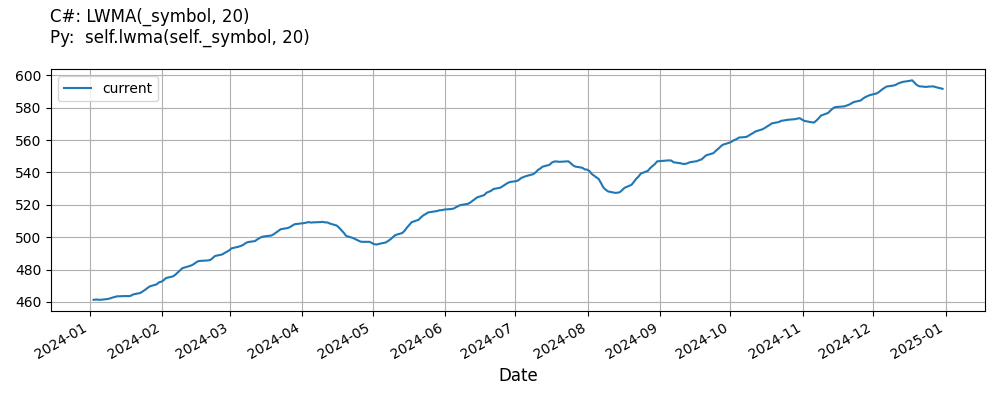# Supported Indicators

## Linear Weighted Moving Average

### Introduction

This indicator represents the traditional Weighted Moving Average indicator. The weight are linearly distributed according to the number of periods in the indicator. For example, a 4 period indicator will have a numerator of (4 * window) + (3 * window) + (2 * window) + window and a denominator of 4 + 3 + 2 + 1 = 10 During the warm up period, IsReady will return False, but the LWMA will still be computed correctly because the denominator will be the minimum of Samples factorial or Size factorial and the computation iterates over that minimum value. The RollingWindow of inputs is created when the indicator is created. A RollingWindow of LWMAs is not saved. That is up to the caller.

To view the implementation of this indicator, see the LEAN GitHub repository.

### Using LWMA Indicator

To create an automatic indicators for LinearWeightedMovingAverage, call the LWMA helper method from the QCAlgorithm class. The LWMA method creates a LinearWeightedMovingAverage object, hooks it up for automatic updates, and returns it so you can used it in your algorithm. In most cases, you should call the helper method in the Initialize method.

public class LinearWeightedMovingAverageAlgorithm : QCAlgorithm
{
private Symbol _symbol;
private LinearWeightedMovingAverage _lwma;

public override void Initialize()
{
_lwma = LWMA(_symbol, 20);
}

public override void OnData(Slice data)
{
{
// The current value of _lwma is represented by itself (_lwma)
// or _lwma.Current.Value
Plot("LinearWeightedMovingAverage", "lwma", _lwma);

}
}
}
class LinearWeightedMovingAverageAlgorithm(QCAlgorithm):
def Initialize(self) -> None:
self.lwma = self.LWMA(self.symbol, 20)

def OnData(self, slice: Slice) -> None:
# The current value of self.lwma is represented by self.lwma.Current.Value
self.Plot("LinearWeightedMovingAverage", "lwma", self.lwma.Current.Value)



The following reference table describes the LWMA method:

### LWMA()1/1

            LinearWeightedMovingAverage QuantConnect.Algorithm.QCAlgorithm.LWMA (
Symbol                           symbol,
Int32                            period,
*Nullable<Resolution>      resolution,
*Func<IBaseData, Decimal>  selector
)


Creates a new LinearWeightedMovingAverage indicator. This indicator will linearly distribute the weights across the periods.

If you don't provide a resolution, it defaults to the security resolution. If you provide a resolution, it must be greater than or equal to the resolution of the security. For instance, if you subscribe to hourly data for a security, you should update its indicator with data that spans 1 hour or longer.

You can manually create a LinearWeightedMovingAverage indicator, so it doesn't automatically update. Manual indicators let you update their values with any data you choose.

Updating your indicator manually enables you to control when the indicator is updated and what data you use to update it. To manually update the indicator, call the Update method with time/number pair, or an IndicatorDataPoint. The indicator will only be ready after you prime it with enough data.

public class LinearWeightedMovingAverageAlgorithm : QCAlgorithm
{
private Symbol _symbol;
private LinearWeightedMovingAverage _lwma;

public override void Initialize()
{
_lwma = new LinearWeightedMovingAverage(20);
}

public override void OnData(Slice data)
{
if (data.Bars.TryGeValue(_symbol, out var bar))
{
_lwma.Update(bar.EndTime, bar.Close);
}

{
// The current value of _lwma is represented by itself (_lwma)
// or _lwma.Current.Value
Plot("LinearWeightedMovingAverage", "lwma", _lwma);

}
}
}
class LinearWeightedMovingAverageAlgorithm(QCAlgorithm):
def Initialize(self) -> None:
self.lwma = LinearWeightedMovingAverage(20)

def OnData(self, slice: Slice) -> None:
bar = slice.Bars.get(self.symbol)
if bar:
self.lwma.Update(bar.EndTime, bar.Close)

# The current value of self.lwma is represented by self.lwma.Current.Value
self.Plot("LinearWeightedMovingAverage", "lwma", self.lwma.Current.Value)



To register a manual indicator for automatic updates with the security data, call the RegisterIndicator method.

public class LinearWeightedMovingAverageAlgorithm : QCAlgorithm
{
private Symbol _symbol;
private LinearWeightedMovingAverage _lwma;

public override void Initialize()
{
_lwma = new LinearWeightedMovingAverage(20);
RegisterIndicator(_symbol, _lwma, Resolution.Daily);
}

public override void OnData(Slice data)
{
{
// The current value of _lwma is represented by itself (_lwma)
// or _lwma.Current.Value
Plot("LinearWeightedMovingAverage", "lwma", _lwma);

}
}
}
class LinearWeightedMovingAverageAlgorithm(QCAlgorithm):
def Initialize(self) -> None:
self.lwma = LinearWeightedMovingAverage(20)
self.RegisterIndicator(self.symbol, self.lwma, Resolution.Daily)

def OnData(self, slice: Slice) -> None:
# The current value of self.lwma is represented by self.lwma.Current.Value
self.Plot("LinearWeightedMovingAverage", "lwma", self.lwma.Current.Value)



The following reference table describes the LinearWeightedMovingAverage constructor:

### LinearWeightedMovingAverage()1/2

            LinearWeightedMovingAverage QuantConnect.Indicators.LinearWeightedMovingAverage (
string  name,
int     period
)


Initializes a new instance of the LinearWeightedMovingAverage class with the specified name and period.

### LinearWeightedMovingAverage()2/2

            LinearWeightedMovingAverage QuantConnect.Indicators.LinearWeightedMovingAverage (
int  period
)


Initializes a new instance of the LinearWeightedMovingAverage class with the default name and period.

### Visualization

The following image shows plot values of selected properties of LinearWeightedMovingAverage using the plotly library.You can also see our Videos. You can also get in touch with us via Discord.